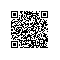# Spark技术内幕：究竟什么是RDD

RDD是Spark最基本，也是最根本的数据抽象。http://www.cs.berkeley.edu/~matei/papers/2012/nsdi_spark.pdf 是关于RDD的论文。如果觉得英文阅读太费时间，可以看这篇译文：http://shiyanjun.cn/archives/744.html

RDD不需要物化。RDD含有如何从其他RDD衍生（即计算）出本RDD的相关信息（即Lineage），据此可以从物理存储的数据计算出相应的RDD分区。

Internally, each RDD is characterized by five main properties:
*
*  - A list of partitions
*  - A function for computing each split
*  - A list of dependencies on other RDDs
*  - Optionally, a Partitioner for key-value RDDs (e.g. to say that the RDD is hash-partitioned)
*  - Optionally, a list of preferred locations to compute each split on (e.g. block locations for
*    an HDFS file)

1. 一组分片（partition），即数据集的基本组成单位
2. 一个计算每个分片的函数
3. 对parent RDD的依赖，这个依赖描述了RDD之间的lineage
4. 对于key-value的RDD，一个Partitioner
5. 一个列表，存储存取每个partition的preferred位置。对于一个HDFS文件来说，存储每个partition所在的块的位置。

RDD支持两种操作：转换（transformation从现有的数据集创建一个新的数据集；而动作（actions）在数据集上运行计算后，返回一个值给驱动程序。 例如，map就是一种转换，它将数据集每一个元素都传递给函数，并返回一个新的分布数据集表示结果。另一方面，reduce是一种动作，通过一些函数将所有的元素叠加起来，并将最终结果返回给Driver程序。（不过还有一个并行的reduceByKey，能返回一个分布式数据集）

Spark中的所有转换都是惰性的，也就是说，他们并不会直接计算结果。相反的，它们只是记住应用到基础数据集（例如一个文件）上的这些转换动作。只有当发生一个要求返回结果给Driver的动作时，这些转换才会真正运行。这个设计让Spark更加有效率的运行。例如，我们可以实现：通过map创建的一个新数据集，并在reduce中使用，最终只返回reduce的结果给driver，而不是整个大的新数据集。

filter(f : T ) Bool) : RDD[T] ) RDD[T]
flatMap(f : T ) Seq[U]) : RDD[T] ) RDD[U]
sample(fraction : Float) : RDD[T] ) RDD[T] (Deterministic sampling)
groupByKey() : RDD[(K, V)] ) RDD[(K, Seq[V])]
reduceByKey(f : (V; V) ) V) : RDD[(K, V)] ) RDD[(K, V)]
union() : (RDD[T]; RDD[T]) ) RDD[T]
join() : (RDD[(K, V)]; RDD[(K, W)]) ) RDD[(K, (V, W))]
cogroup() : (RDD[(K, V)]; RDD[(K, W)]) ) RDD[(K, (Seq[V], Seq[W]))]
crossProduct() : (RDD[T]; RDD[U]) ) RDD[(T, U)]
mapValues(f : V ) W) : RDD[(K, V)] ) RDD[(K, W)] (Preserves partitioning)
sort(c : Comparator[K]) : RDD[(K, V)] ) RDD[(K, V)]
partitionBy(p : Partitioner[K]) : RDD[(K, V)] ) RDD[(K, V)]

collect() : RDD[T] ) Seq[T]
reduce(f : (T; T) ) T) : RDD[T] ) T
lookup(k : K) : RDD[(K, V)] ) Seq[V] (On hash/range partitioned RDDs)
save(path : String) : Outputs RDD to a storage system, e.g., HDFS

# 从一个例子开始

lines = spark.textFile("hdfs://...")  // lines is a org.apache.spark.rdd.MappedRDD
errors = lines.filter(_.startsWith("ERROR")) // errors is a org.apache.spark.rdd.FilteredRDD
errors.cache() // persist 到内存中
errors.count() // 触发action，计算errors有多少个，即ERROR的多少行
// Count errors mentioning MySQL:
errors.filter(_.contains("MySQL")).count()
// Return the time fields of errors mentioning
// HDFS as an array (assuming time is field
// number 3 in a tab-separated format):
errors.filter(_.contains("HDFS"))
.map(_.split('\t')(3))
.collect()

spark是一个org.apache.spark.SparkContext的实例，基本上spark的应用都是以定义一个SparkContext开始的。textFile的定义如下：

 /**
* Read a text file from HDFS, a local file system (available on all nodes), or any
* Hadoop-supported file system URI, and return it as an RDD of Strings.
*/
def textFile(path: String, minPartitions: Int = defaultMinPartitions): RDD[String] = {
minPartitions).map(pair => pair._2.toString).setName(path)
}

  /**
* Return a new RDD by applying a function to all elements of this RDD.
*/
def map[U: ClassTag](f: T => U): RDD[U] = new MappedRDD(this, sc.clean(f))

errors.cache()并不会立即执行，它的作用是在RDD的计算完成后，将结果cache起来，以供以后的计算使用，这样的话可以加快以后运算的速度。

errors.count() 就触发了一个action，这个时候就需要向集群提交job了：

 /**
* Return the number of elements in the RDD.
*/
def count(): Long = sc.runJob(this, Utils.getIteratorSize _).sum使用钉钉扫一扫加入圈子
+ 订阅